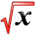# Approximate IntegrationThis article is part of the MathHelp Tutoring Wiki

Question: We need to approximate integral from a to b (f(x)*dx)

A. Midpoint Rule

Step1: Divide interval [a,b] in "n" identical subintervals.

delta x= (b-a)/n

X1*=(Xo+X1)/2, X2*=(X1+X2)/2, Xi*=(Xi-1+Xi)/2

so

X1=X0+deltaX

X2=X1+deltaX

integration from a to b (f(x)*dx)= deltaX* f(X1*)+deltaX*f(X2*)+...+deltaX*f(Xn*)

=Mn=deltaX*(f(X1*)+f(X2*)+f(X3*)+...+f(Xn*))

Error in midpoint rule approximation

Note: integration from a to b (f(x)*dx)=exact result

Mn is the approximation of integration from a to b f(x)*dx

Em=|Mn-integration from a to b (f(x)*dx)

Theorem: If |f (x)|<=k for any x, a<=x<=b then Em<=k(b-a)3/(24M2)

Example: Write M4 for integration from 0 to pi/2 (cosx*dx) delta x=pi/2/4=pi/8

X0=a=0,

X1=0+pi/8, X1*= (X0+X1)/2=pi/16

X2=0+2*pi/8, X2*=(X1+X2)/2=3pi/16

X3=0+3pi/8, X3*=(X2+X3)/2=5pi/16

X4=pi/2, X4*=7pi/16

M4= pi/8*(f(x1*)+f(x2*)+f(x3*)+f(x4*))

=pi/8*(cosp/16+cos3pi/16+cos 5pi/16+cos 7pi/16)'